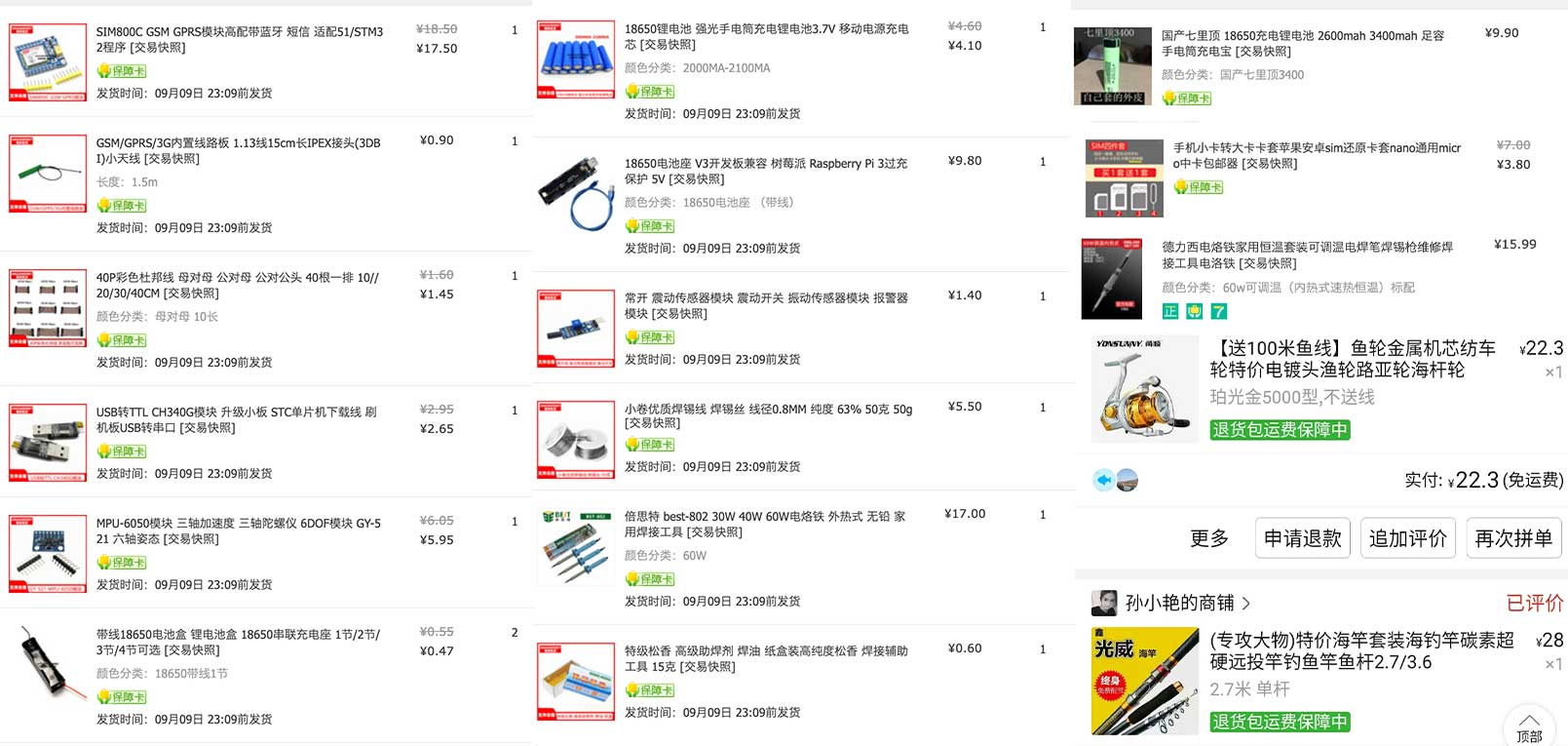# 钓鱼的感想(三): 自动钓大鱼

Oct 03, 2021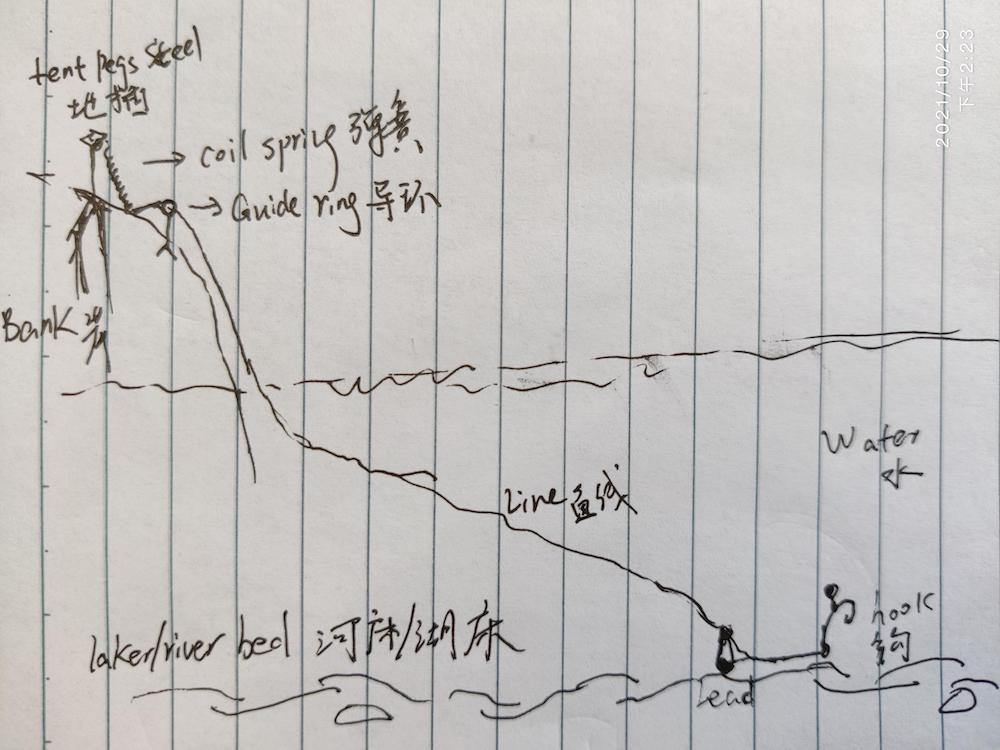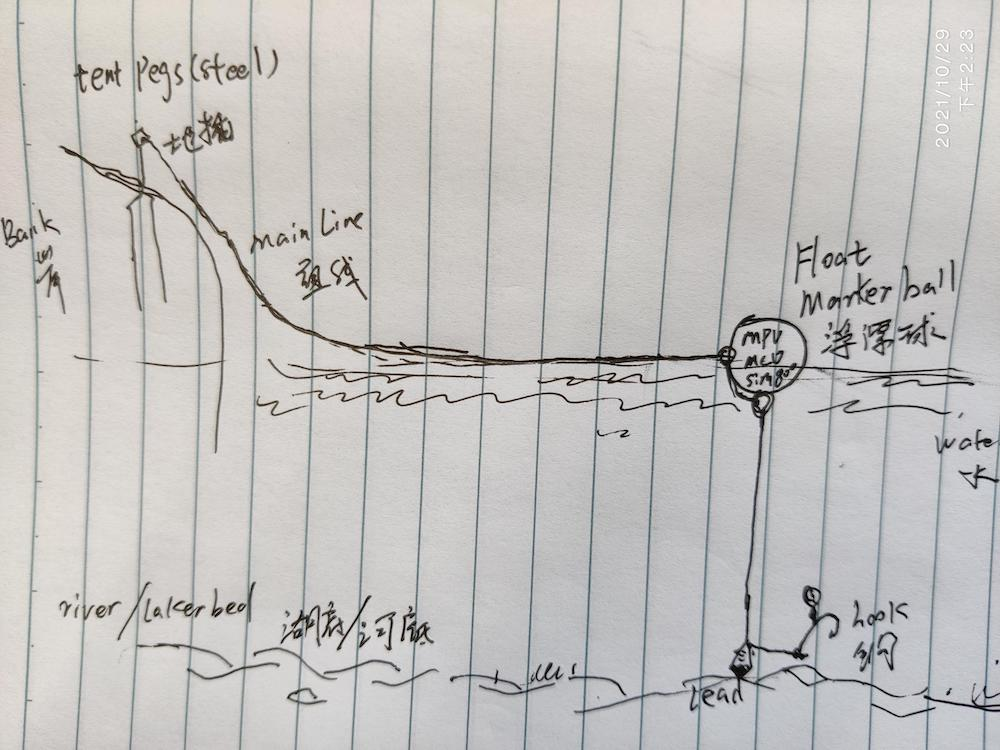• 装置在岸上，不用考虑防水
• 抛竿流程不受影响， 正常抛竿即可
• 不用考虑风浪，也不用考虑暴晒散热
• 一旦出现挂底，损失比较小，岸上的装置等不受影响

• 隐蔽性不高，目标还是有点大， 容易被过路的其他人和钓鱼人发现拿走，不好隐藏
• 中鱼信号的准确度有所欠缺，要求鱼线需拉直紧绷，能及时在长距离传递信号
• 受岸边地形限制 - 必须有合适的障碍物来伪装隐蔽

• 浮漂垂直在钩子的上方，鱼线距离比较短，中鱼信号的准确度高
• 隐蔽性强，因为浮漂在30-50米之外，其他人无法简单地够着，所以不容易被偷窃
• 不受岸边地形限制 - 地插可以在水里也可以是岸上，目标小

• 浮漂材质要求比较高，需要既能有好的密闭性能防水也能方便打开，没有类似的现成既有的商品，需要DIY和不断试验
• 风浪 - 需要在大风浪下，水面有波浪的情况下，保持检测的准确度，同时大风浪意味着需要更大的铅坠来保持定位，这样对鱼竿、渔轮都有更高的要求
• 散热 - 在密闭性要求高的情况下，夏天中午太阳直射和水面的反光，会导致浮漂内部温度升高，是否电子元器件可以正常工作？
• 抛竿难度增加，毕竟浮漂本身也有很大的重量加上大的铅坠
• 一旦线断开，极有可能导致浮漂丢失，这样里面的装置都会丢失，损失比较大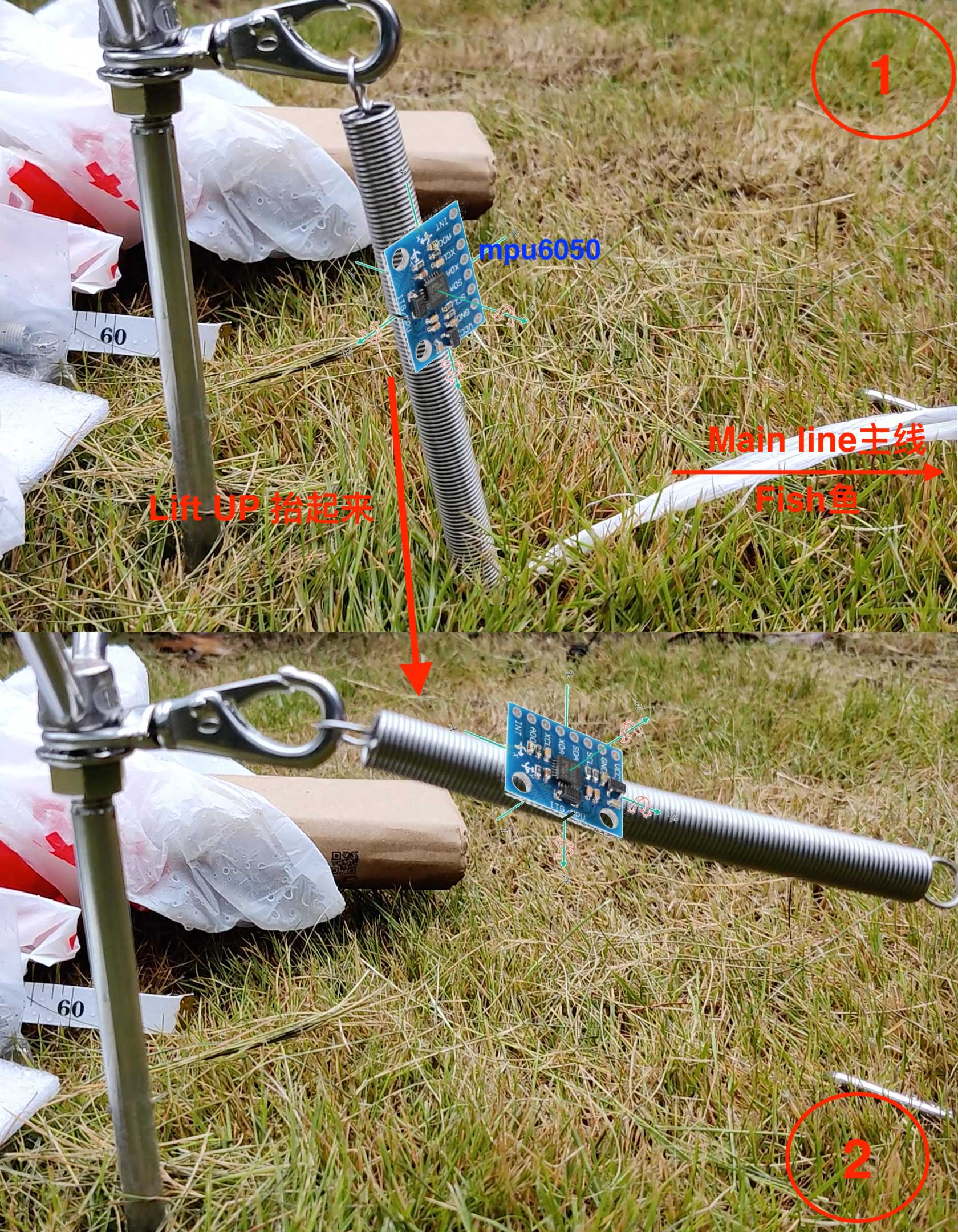## ESP8266 微控制单元

MCU，微控制单元也叫单片微型计算机， 或者简单点说单片机，是装置的核心模块。单片机，简单理解为微型电脑，特点是体积小，功耗低，有不同的通信模式协议来接入不同的外围设备，比如打印机、显示器等等，互相交换数据。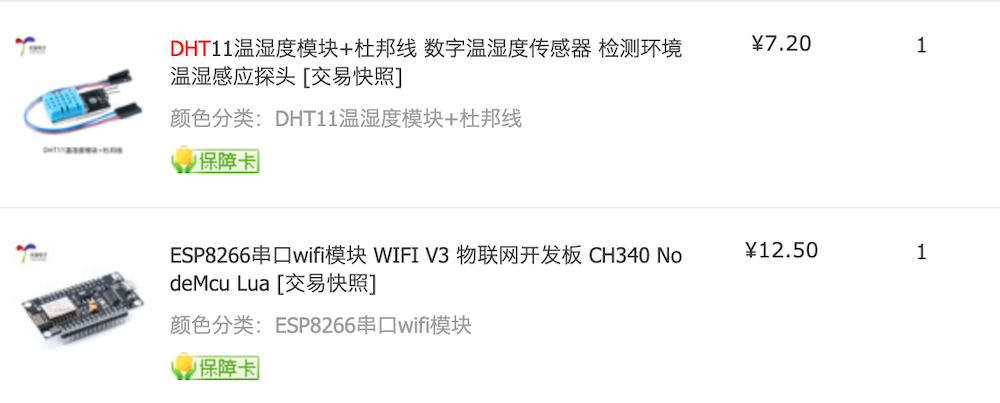NodeMCU 是一款开源的物联网开发平台，支持各种固件系统，比如淘宝上提到NodeMCU Lua V3，也就是基于脚本语言Lua的NodeMCU，还有其他比较流行的MicroPython(Python)、Mongoose OS(NodeJS)等等。这些在系统底层的基础上加了一个解释器，可以直接修改代码而快速的调试，而不用每次改动都编译为底层汇编字节码并上传Flash，后者这样开发调试的速度实在太慢了。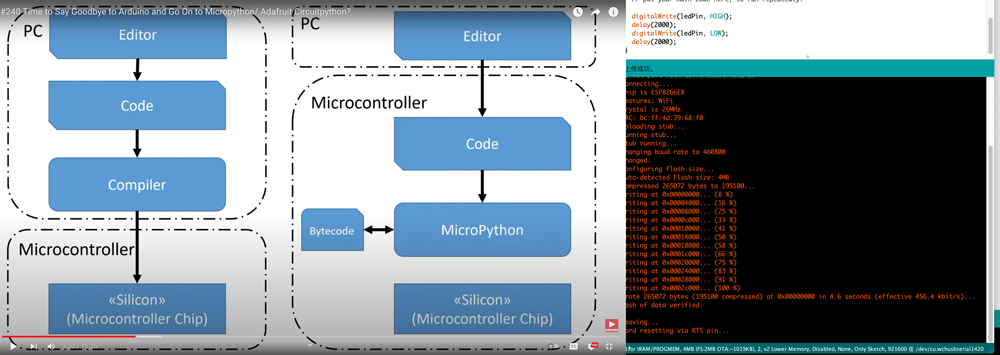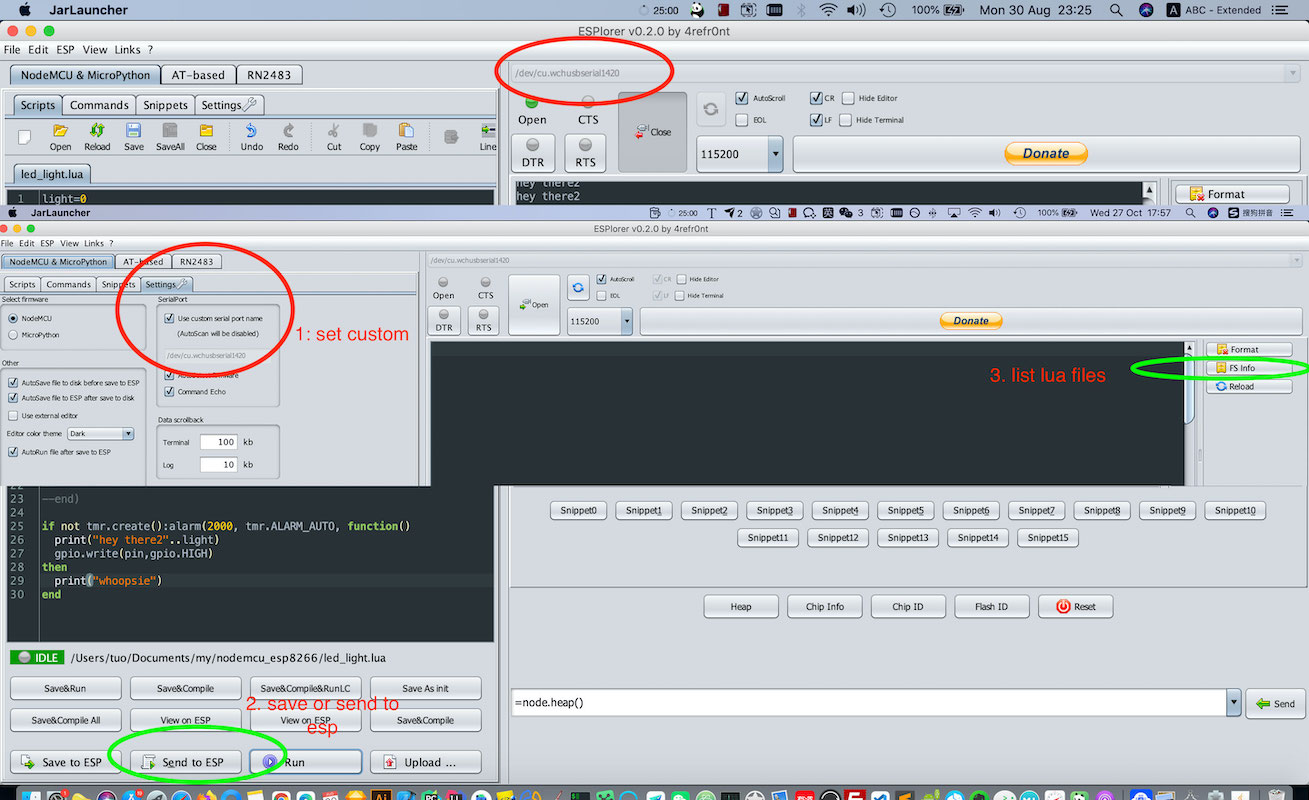NodeMCU还是非常好上手的，特别是自带了wifi模块，可以尝试从简单点亮板载LED到读取外设传感器比如测量温湿度的DHT11温湿度模块（7块钱）的值并通过WIFI上传到服务器上，对照着nodemcu的文档和教程，一步步摸索和熟悉下基础的单片机知识。

## MPU6050 - 角度加速度六轴传感器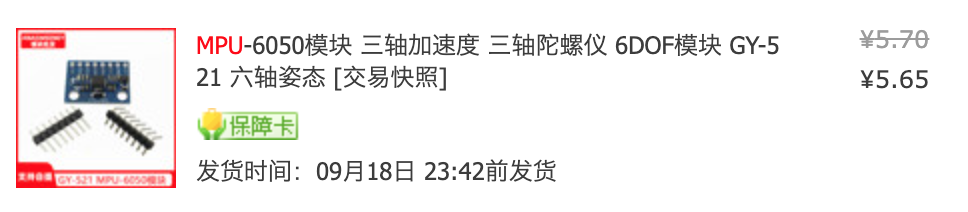MPU6050传感器是一个集成了6轴运动跟踪装置的模块，分别是3轴陀螺仪和3轴加速度计，同时集成了数字运动处理器和温度传感器。通过I2C总线，他还可以接受来自其他传感器的输入，如3轴磁力计或压力传感器，因此如果将MPU6050与外部的3轴磁力计连接起来，它就可以提供完整的9轴输出了。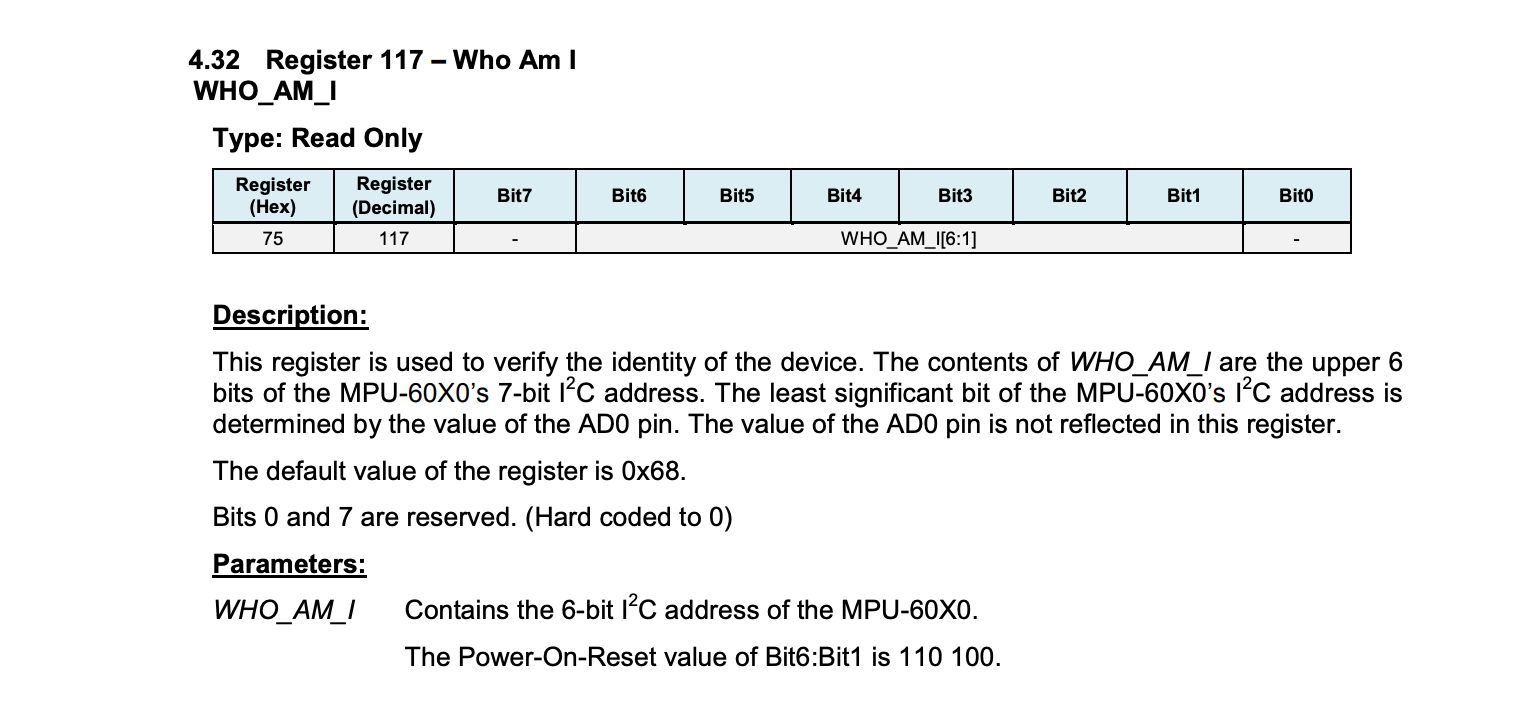``````-- https://invensense.tdk.com/wp-content/uploads/2015/02/MPU-6000-Register-Map1.pdf
id  = 0
scl = 7
sda = 6
AccelScaleFactor = 4096;  --8g/s
GyroScaleFactor = 65.5; -- ± 500 °/s

MPU6050_REGISTER_SMPLRT_DIV   =  0x19
MPU6050_REGISTER_USER_CTRL    =  0x6A
MPU6050_REGISTER_PWR_MGMT_1   =  0x6B
MPU6050_REGISTER_PWR_MGMT_2   =  0x6C
MPU6050_REGISTER_CONFIG       =  0x1A
MPU6050_REGISTER_GYRO_CONFIG  =  0x1B
MPU6050_REGISTER_ACCEL_CONFIG =  0x1C
MPU6050_REGISTER_FIFO_EN      =  0x23
MPU6050_REGISTER_INT_ENABLE   =  0x38
MPU6050_REGISTER_ACCEL_XOUT_H =  0x3B
MPU6050_REGISTER_SIGNAL_PATH_RESET  = 0x68

i2c.start(id)       -- send start condition
then
print("write")
i2c.write(id, data)  -- write data to slave
i2c.stop(id)    -- send stop condition
else
print("I2C_Write fails")
end
end

response = 0;
i2c.start(id)       -- send start condition
then
i2c.stop(id)    -- send stop condition
i2c.start(id)   -- send start condition
i2c.stop(id)    -- send stop condition
return response
else
end
return response
end

function unsignTosigned16bit(num)   -- convert unsigned 16-bit no. to signed 16-bit no.
if num > 32768 then
num = num - 65536
end
return num
end
function MPU6050_Init() --configure MPU6050
tmr.delay(150000) -- delay for 150 ms
I2C_Write(MPU6050SlaveAddress, MPU6050_REGISTER_GYRO_CONFIG, 0x08)-- set +/-500 degree/second full scale
I2C_Write(MPU6050SlaveAddress, MPU6050_REGISTER_ACCEL_CONFIG, 0x10)-- set +/- 8g full scale
end
i2c.setup(id, sda, scl, i2c.SLOW)   -- initialize i2c
MPU6050_Init()
tmr.delay(1000)]

while true do   --read and print accelero, gyro and temperature value
AccelX = unsignTosigned16bit((bit.bor(bit.lshift(string.byte(data, 1), 8), string.byte(data, 2))))
AccelY = unsignTosigned16bit((bit.bor(bit.lshift(string.byte(data, 3), 8), string.byte(data, 4))))
AccelZ = unsignTosigned16bit((bit.bor(bit.lshift(string.byte(data, 5), 8), string.byte(data, 6))))
Temperature = unsignTosigned16bit(bit.bor(bit.lshift(string.byte(data,7), 8), string.byte(data,8)))
GyroX = unsignTosigned16bit((bit.bor(bit.lshift(string.byte(data, 9), 8), string.byte(data, 10))))
GyroY = unsignTosigned16bit((bit.bor(bit.lshift(string.byte(data, 11), 8), string.byte(data, 12))))
GyroZ = unsignTosigned16bit((bit.bor(bit.lshift(string.byte(data, 13), 8), string.byte(data, 14))))

-- ACC in g unit, Temp  in degree/celcius, Gyro in degree/celcius
AccelX = AccelX/AccelScaleFactor   -- divide each with their sensitivity scale factor
AccelY = AccelY/AccelScaleFactor
AccelZ = AccelZ/AccelScaleFactor
Temperature = Temperature/340.0+36.53-- temperature formula
GyroX = GyroX/GyroScaleFactor
GyroY = GyroY/GyroScaleFactor
GyroZ = GyroZ/GyroScaleFactor

print(string.format("TUO-Ax:%.3g Ay:%.3g Az:%.3g T:%.3g Gx:%.3g Gy:%.3g Gz:%.3g",
AccelX, AccelY, AccelZ, Temperature, GyroX, GyroY, GyroZ))
tmr.delay(100000)   -- 100ms timer delay
end
``````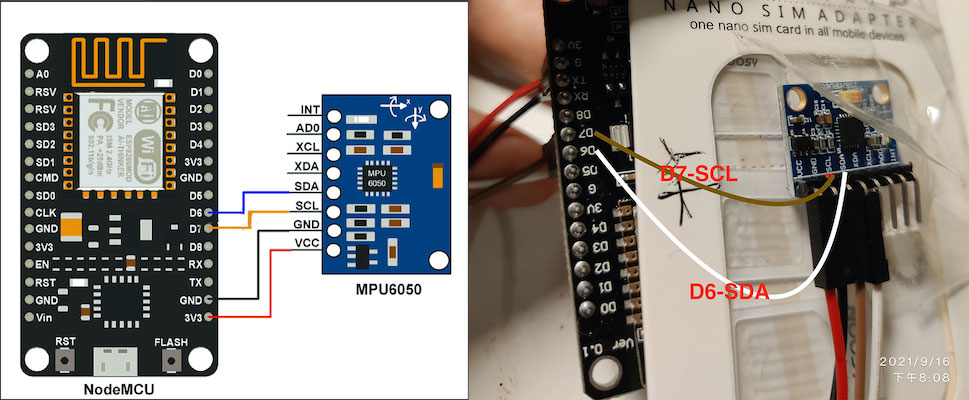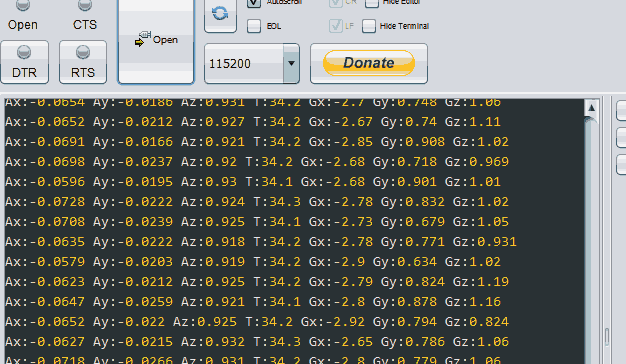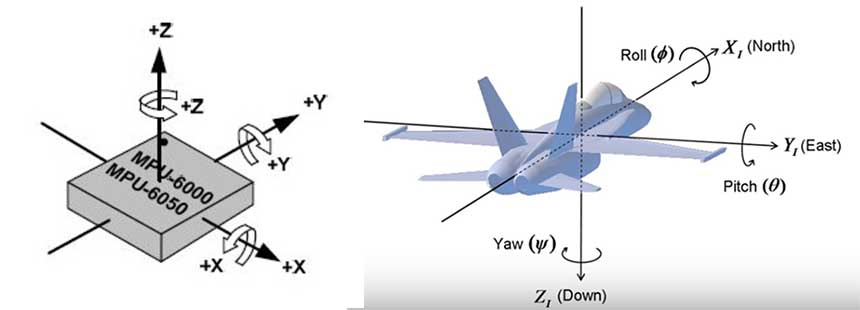``````//source: http://www.brokking.net/imu.html MPU-6050 6dof IMU for auto-leveling multicopters

//Gyro angle calculations: 0.0000611 = 1 / (250Hz / 65.5)
angle_pitch += gyro_x * 0.0000611; //Calculate the traveled pitch angle and add this to the angle_pitch variable
angle_roll += gyro_y * 0.0000611;  //Calculate the traveled roll angle and add this to the angle_roll variable

//0.000001066 = 0.0000611 * (3.142(PI) / 180degr) The Arduino sin function is in radians
angle_pitch += angle_roll * sin(gyro_z * 0.000001066); //If the IMU has yawed transfer the roll angle to the pitch angel
angle_roll -= angle_pitch * sin(gyro_z * 0.000001066); //If the IMU has yawed transfer the pitch angle to the roll angel

//Accelerometer angle calculations
acc_total_vector = sqrt((acc_x * acc_x) + (acc_y * acc_y) + (acc_z * acc_z)); //Calculate the total accelerometer vector
//57.296 = 1 / (3.142 / 180) The Arduino asin function is in radians
angle_pitch_acc = asin((float)acc_y / acc_total_vector) * 57.296; //Calculate the pitch angle
angle_roll_acc = asin((float)acc_x / acc_total_vector) * -57.296; //Calculate the roll angle

//Place the MPU-6050 spirit level and note the values in the following two lines for calibration
angle_pitch_acc -= 0.0; //Accelerometer calibration value for pitch
angle_roll_acc -= 0.0;  //Accelerometer calibration value for roll

if (set_gyro_angles){  //If the IMU is already started
angle_pitch = angle_pitch * 0.9996 + angle_pitch_acc * 0.0004; //Correct the drift of the gyro pitch angle with the accelerometer pitch angle
angle_roll = angle_roll * 0.9996 + angle_roll_acc * 0.0004;  //Correct the drift of the gyro roll angle with the accelerometer roll angle
}else{  //At first start
angle_pitch = angle_pitch_acc; //Set the gyro pitch angle equal to the accelerometer pitch angle
angle_roll = angle_roll_acc;   //Set the gyro roll angle equal to the accelerometer roll angle
set_gyro_angles = true;        //Set the IMU started flag
}
//To dampen the pitch and roll angles a complementary filter is used
angle_pitch_output = angle_pitch_output * 0.9 + angle_pitch * 0.1; //Take 90% of the output pitch value and add 10% of the raw pitch value
angle_roll_output = angle_roll_output * 0.9 + angle_roll * 0.1;    //Take 90% of the output roll value and add 10% of the raw roll value
``````

## SIM800C - 无线通信模块

G(GPRS)→E(EDGE)→3G(WCDMA)→ H(HSPA)→H+(HSPA+)→4G/LTE(LTE-FDD/TDD)

G,E属于2G，3G,H属于3G，H+,4G/LTE属于4G，速度依次增快。 这几种网络使用感受上的最大区别当然就是速度和可以传输数据的类型不同，比如2G主要是语音业务，2.5G就可以传送数据业务，3G达到快速数据业务传输，H使数据速率更高，LTE提高了容量减小了延迟，实现全数据网络等等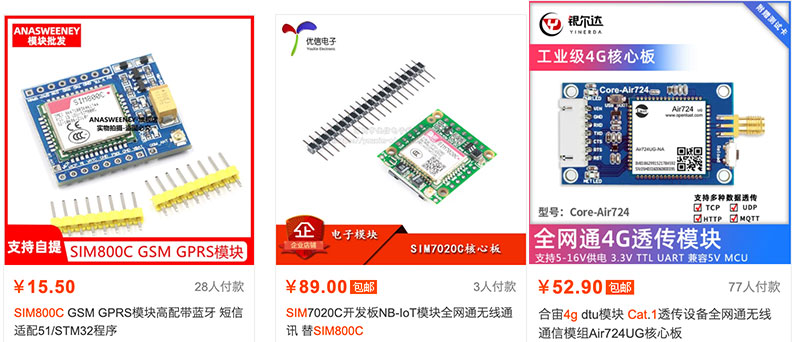SIM800C可以通过AT指令发送命令，可以先连接电脑，调试一下基本的指令。这个时候需要一个USB-TTL调试模块、一个手机sim卡、可能还需要一个Nano卡槽和Sim800c模块。SIM800C插好手机卡，然后使用USB-TTL连接SIM800C然后插入电脑供电，观察到电源指示灯亮起之后，用跳线帽或者杜邦线将PWX和GND短接，给到一个低电平给PWX来出发启动模块，这个时候模块才会开始工作。调试时特别注意下LED闪灯频率，这个可以区分模块的工作状态。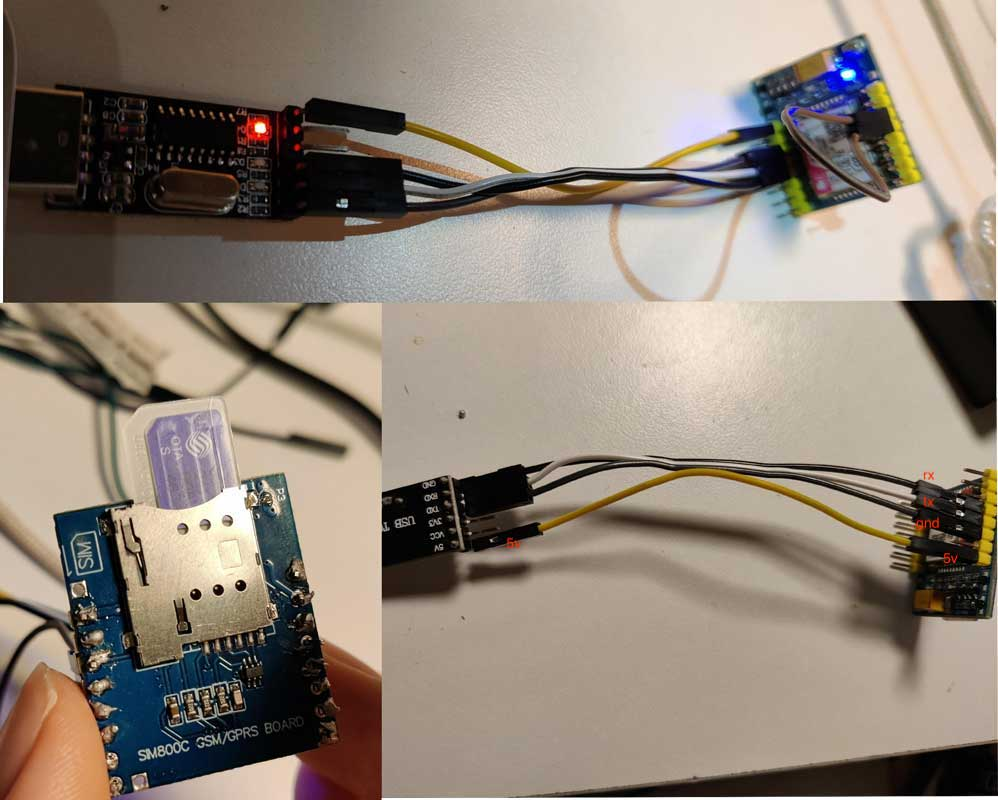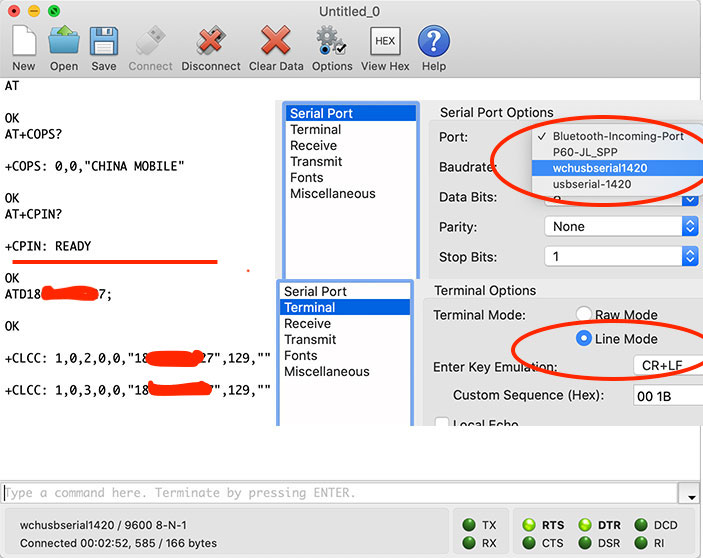SIM800C可以接受哪些AT指令，建议大家参考下它的数据手册，但是中文的数据手册在线的没有找到，只有这个勉强能用《SIM800C系列用户使用手册》，一般来说你购买的淘宝店家应该会给到你一份手册。我们这里用到的包括打电话和每个一分钟发送一次MPU6050采集的角速度加速度温度信息到服务器方便后续排查和调试。下面是连线示意和SIM800代码：

MCU - SIM800C

RX(D3) - TX

TX(D2) - RX

GND <-> GND

VCC <-> VBAT

``````---- Create new software UART with baudrate of 9600, D2 as Tx pin and D3 as Rx pin
function writeCMD(s, cmd)
print("\n"..tostring(tmr.now())..": send cmd", cmd);
s:write(cmd.."\n");
end

function sim_setup()
if not s then
print("\n"..tostring(tmr.now())..": initilized su\n");
s = softuart.setup(9600, 2, 3)
s:on("data", "\n", function(data)
local txt = string.gsub(data, "[\r\n]", "")
local pattern = "^HTTPACTION"
if txt:find(pattern) ~= nil then
print("\n"..tostring(tmr.now())..": RECIEVED HTTP ACTION DONE:", txt)
writeCMD(s, 'AT+HTTPTERM')
--          writeCMD(s, 'AT+SAPBR=0,1')
end
end)
else
print("\n"..tostring(tmr.now())..": existed su\n");
end
end
function sim_send(txt)
writeCMD(s, 'AT+SAPBR=3,1,"Contype","GPRS"')
writeCMD(s, 'AT+SAPBR=3,1,"APN","CMNET"')
writeCMD(s, 'AT+SAPBR=1,1')
writeCMD(s, 'AT+SAPBR=2,1')
writeCMD(s, 'AT+HTTPINIT')
writeCMD(s, 'AT+HTTPPARA="CID",1')
local url = "xxxx.com/api/dashboard?time="..tostring(tmr.now().."&txt="..txt)
writeCMD(s, 'AT+HTTPPARA="URL","'..url..'"')
writeCMD(s, 'AT+HTTPACTION=0')
s:write(0x1a);
end

function sim_call()
writeCMD(s, 'ATD186xxxx5235;')
end
``````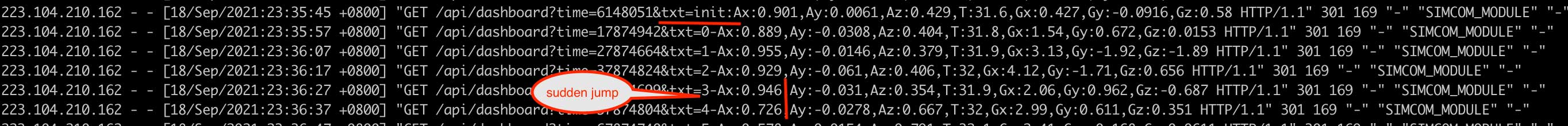### 常见问题 - SIM800自动关机、工作不稳定

SIM800跟ESP8266 MCU通信是通过TTL电平的方式，也就是高电位是1，低电位是0， 这样就可以组合以二进制的方式在双方之间传递数据信息。这就意味着这单片机和Sim800必须有相同的板载电压作为高低压，低电位好理解，都是接地。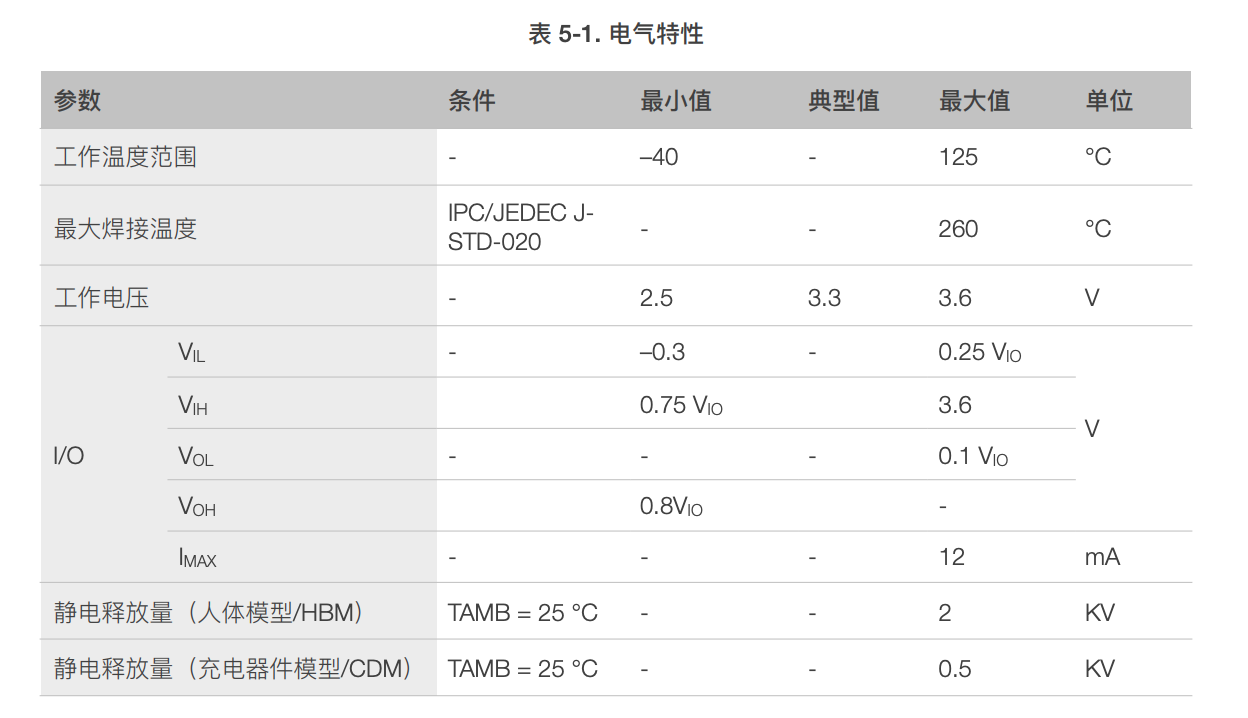4.1

4.1.1

#### 第一个： 5V降压LDO

​ 使用5v的电源，用额定2A的DC-DC降压转换器比如LM2596(2块钱左右，可输出高达3A的电流)，将输出连接到SIM800C的VBAT。

#### 第二个： 使用单独的3.7锂电池（推荐）

​ 技术手册里在章节4.1.1里提到`客户可以直接用3.7V的锂离子电池给模块供电`。这里可以用18650锂电池，2000mAh左右或者往上的大容量功率，能够确保在2A的峰值电流下，还能保持正确的电压范围。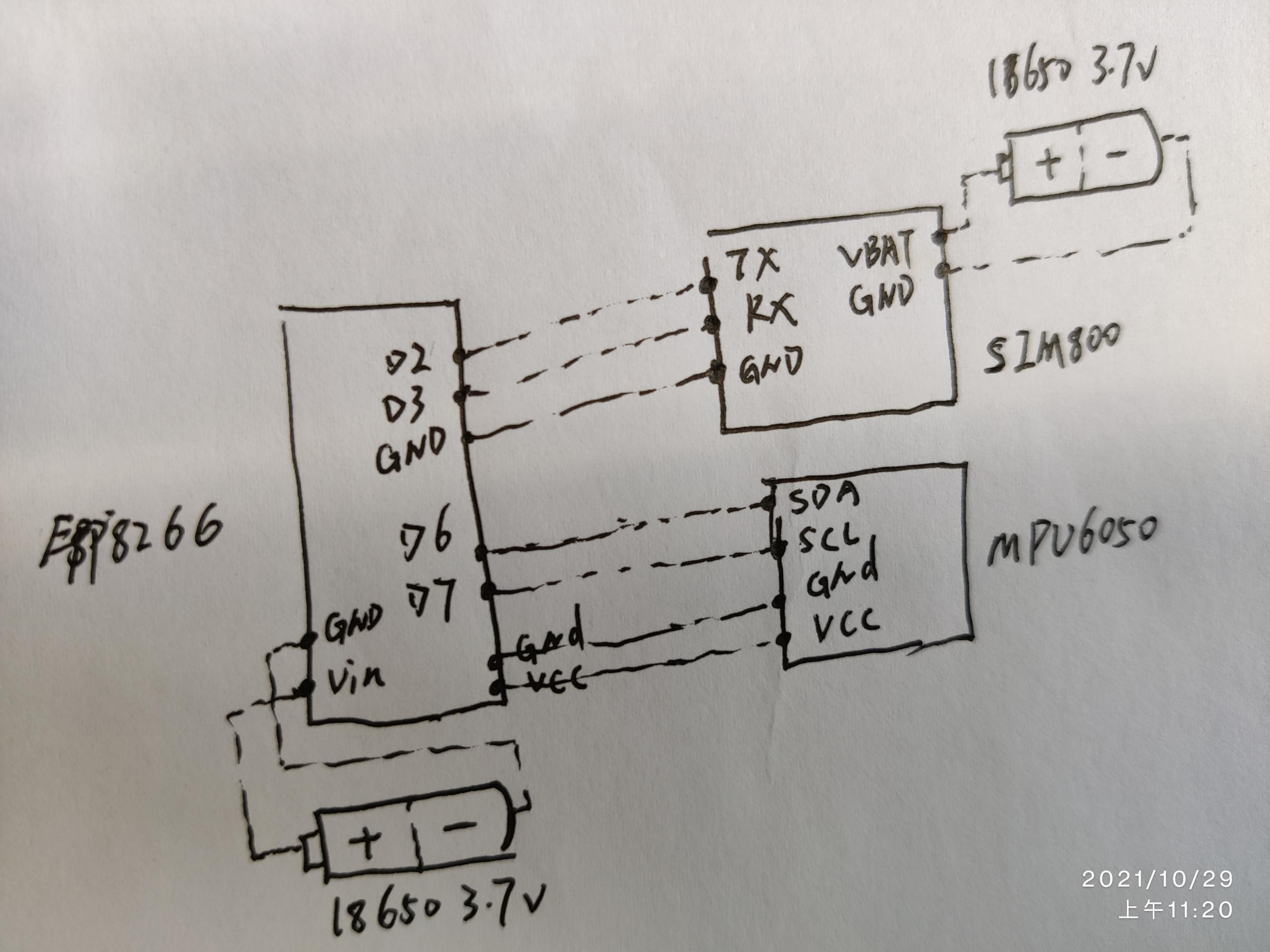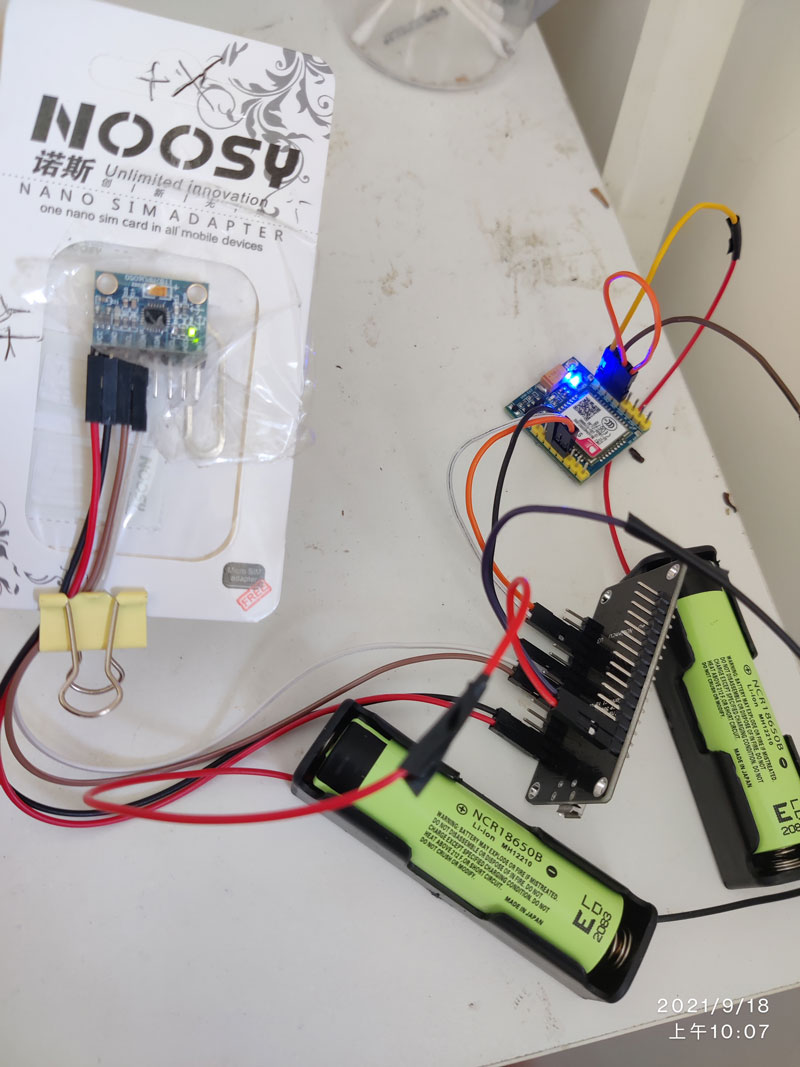### 电池续航可以使用多久？

MPU6050每隔2秒读一次数据，每隔1分钟发送一次HTTP请求，大概2500mA的电池可以支持两天没有问题，这个足以符合我们当初设想的要求。关于电池放电这块，需要了解电池的放电曲线measured discharge curves for 18650 cells ，也就是随着时间输出电压是下降的, 当下降到一定电压时，就无法给模块提供足够的电量。

• 换更大的电池比如3300mA的
• 其他更细的技术的电池比如基于Lipo， 18650是基于Lithium-Ion Lithium-Ion (18650 cells) versus Lipo – which is best?
• 拉长采样时间 - 比如每隔2秒可以是5秒，1分钟可以是5分钟等等
• 中断 - 与其不断去询问数据并计算没有超过阈值，可以用中断的思路，设置好阈值，只有当传感器的对应数值超过阈值时，才会跟通知单片机
• 单片机有低功耗模式 - 可以参考技术手册，根据实际调整

## 实战的问题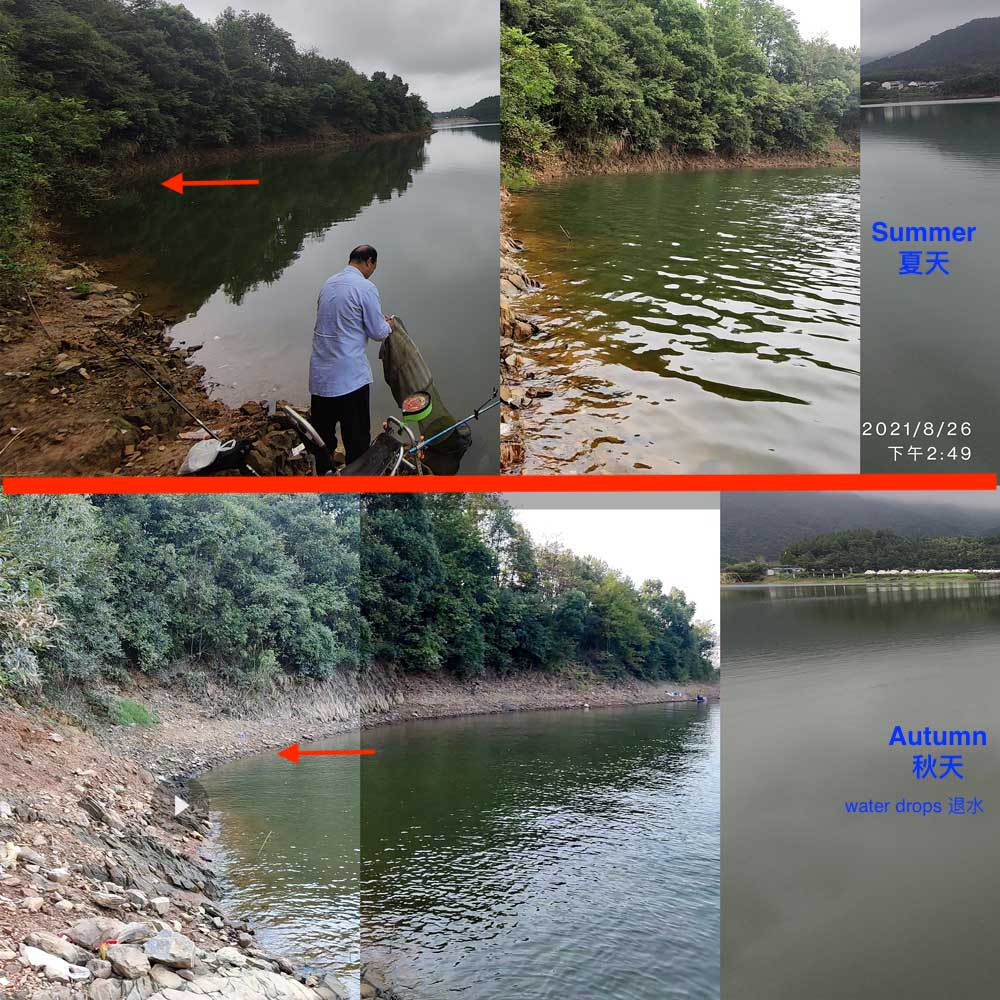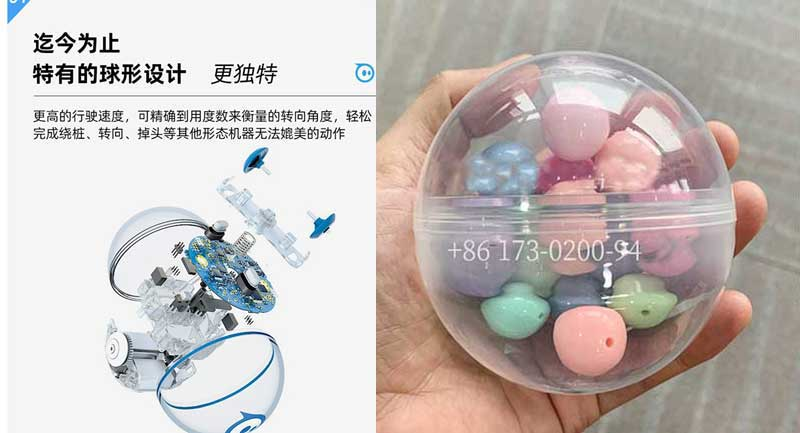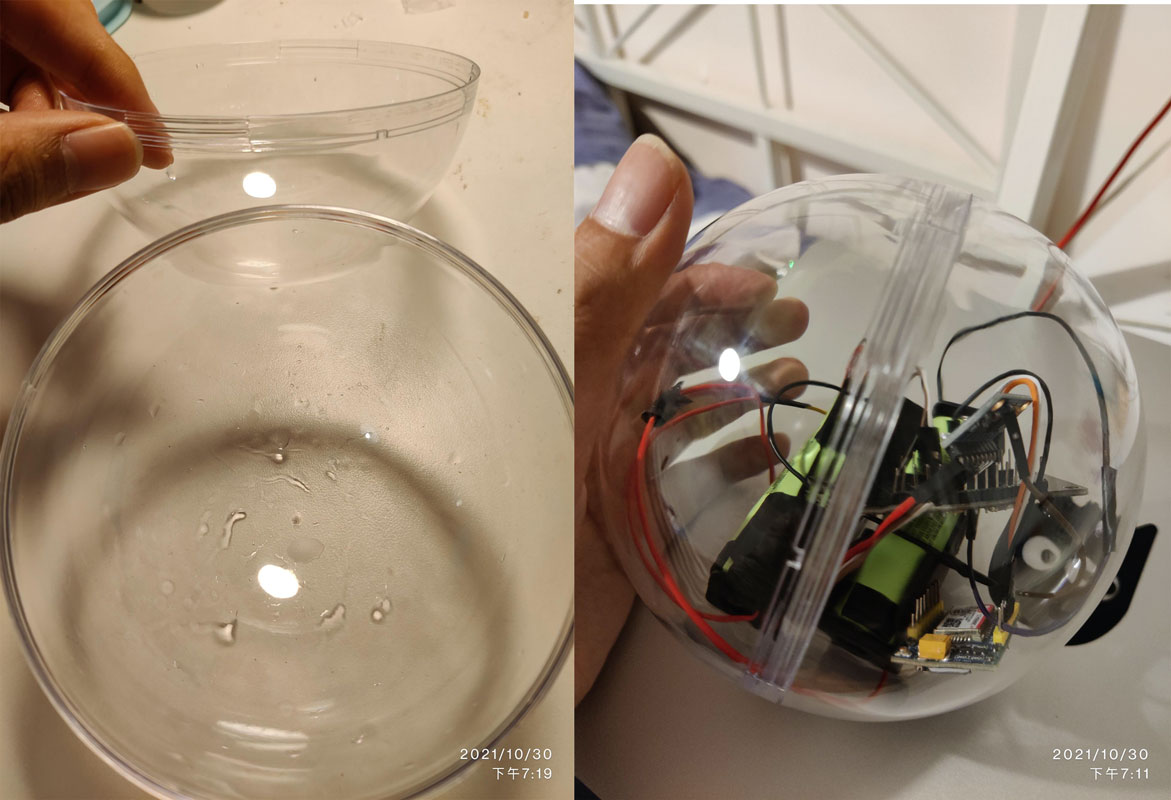## 淘宝购买的部件清单